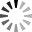# Monte Carlo simulations techniques use for Risk Management

Monte Carlo simulations techniques use for Risk Management💥💥Monte Carlo simulations are a powerful tool used in quantitative analysis to model complex systems with a large number of variables and uncertainties. The technique is named after the famous casino in Monaco, which is known for its games of chance.

⚡️Monte Carlo simulations use random sampling to generate a large number of scenarios, and then calculate the probability of various outcomes. The simulations are especially useful in finance and investing, where there are many variables and uncertainties that can impact investment returns.

💥To use Monte Carlo simulations in finance, investors typically start with a set of assumptions about the market and the economy, such as expected returns, volatility, and correlations among asset classes. They then use these assumptions to generate a large number of potential scenarios, each with a different set of values for these variables.

⚡️For example, an investor might use Monte Carlo simulations to model the potential returns of a portfolio of stocks and bonds. They would start by assuming a certain level of expected returns and volatility for each asset class, and then generate a large number of scenarios with different values for these variables. The simulations might show that there is a high probability of achieving a certain level of return, but also a significant risk of losing money in certain scenarios.

💥Investors can use Monte Carlo simulations to optimize their portfolios by adjusting their asset allocation or risk management strategies based on the results of the simulations. For example, if the simulations show a high risk of significant losses in certain scenarios, the investor may choose to reduce their exposure to those assets or implement a risk management strategy such as stop-loss orders.

⚡️Another common use of Monte Carlo simulations in finance is to model the potential impact of different economic scenarios, such as a recession or inflation. By generating a large number of potential scenarios and analyzing the results, investors can gain insight into the potential risks and opportunities of different market conditions.

💥Monte Carlo simulations are a valuable tool for investors and analysts seeking to model complex financial systems and make informed decisions based on probabilities and risk analysis. However, it is important to remember that Monte Carlo simulations are only as good as the assumptions and data used to generate them, and should be used in conjunction with other analytical and qualitative methods to make well-informed investment decisions.

Here are some examples of how Monte Carlo simulations can be used in different applications:

👉 1. Portfolio Optimization: Monte Carlo simulations can be used to optimize portfolio allocation by generating different simulations of the possible future performance of different asset classes. By using a wide range of possible scenarios, the investor can identify the optimal asset allocation that maximizes return while minimizing risk.

👉 2. Stress Testing: Monte Carlo simulations can be used to stress test a portfolio by modeling the impact of different scenarios on the performance of the portfolio. This can help investors identify potential vulnerabilities and build a more robust portfolio.

👉 3. Option Pricing: Monte Carlo simulations are widely used in option pricing models. By simulating various scenarios, option prices can be calculated by generating an average of the simulated outcomes. This helps investors price options more accurately.

👉 4. VaR (Value at Risk) Analysis: Monte Carlo simulations can be used to calculate the VaR of a portfolio. This involves generating a large number of simulations of future returns and calculating the worst-case loss that could occur at a given level of confidence. This helps investors understand the downside risk of their portfolio and take appropriate risk management measures.

👉 5. Retirement Planning: Monte Carlo simulations can be used to model different scenarios for retirement planning. By simulating different levels of investment returns and inflation rates, investors can determine the probability of meeting their retirement goals and adjust their investment strategy accordingly.

💥💥Overall, Monte Carlo simulations are a versatile tool that can be applied to many different areas of quantitative analysis. By using these simulations, investors can gain a better understanding of the risks associated with different investment strategies and make more informed investment decisions.

Tags:

 Thanks:

Attach files by dragging & dropping, , or pasting from the clipboard.